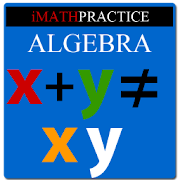# Master Algebra LiteEveryone
137
This is for students in High School/College learning algebra. If you are a beginner in algebra you might be thinking X+Y=XY, Is not it? But it’s not.
The beauty of algebra is, it deals with variables, expressions & equations. You will come to know various formulas.
For example if you know (a+b)^3= a^3+b^3+3a^2b+3ab^2.
You can calculate any number to the powers 2,3,4…in a fraction of seconds.
In the above equation a ,b are variables. So you can calculate (1.034)^3 also using that formula. Just feed a=1& b=.034
IMathPractice Algebra's 3 steps method of teaching has sections like Tutorial, Practice Skills, Practice Test & Algebra Challenge. Under tutorial it teaches you.

Numbers
Types of Number like real number, integer, negative number, complex number
Addition, Subtraction, Multiplication & Division of Real Number
Addition, Subtraction, Multiplication & Division of Negative Number
Addition, Subtraction, Multiplication & Division of Complex Number
Properties of Number

Ratio & Proportion

Integer exponents/real /rational exponents

What is Monomial/binomial/polynomial?
Addition, Subtraction, Multiplication, Division of polynomials
Factoring polynomial

What is variable, expression & equation?
Equation with radical, Absolute value of equation
Rational Expression;
Addition, Subtraction, Multiplication, Division of rational expression

What’s Inequality?
Linear, Polynomial &Rational Inequalities
Absolute value of Inequality

Under Practice Skills, You will be able to practice all the above learned skills with help.
There are answer & steps to get the answer for each question.

Under Practice Test, You will be able to practice all the skills in specified timed environment. There is a timer. You need to finish within that time.

Under Algebra Challenge You will be prepared to compete with others. It contains 50 questions & time allotted is 1 hour.

There are around 210 questions to practice for in lite version.

What's new in version 1.3?
Bug reported in comment section has been removed.

Note:Currently the app is in English only. We are working on translation to other languages. Please don't rate 1 only because it's not in your language.You can send your request to developer.
Collapse

## Reviews

Review Policy
3.6
137 total
5
4
3
2
1

Updated
November 9, 2013
Size
524k
Installs
50,000+
Current Version
1.3
Requires Android
2.1 and up
Content Rating
Everyone
Permissions
Offered By
ongolearn.com
Developer
3996 Fleming Street Vancouver,BC Canada V5N 3W3